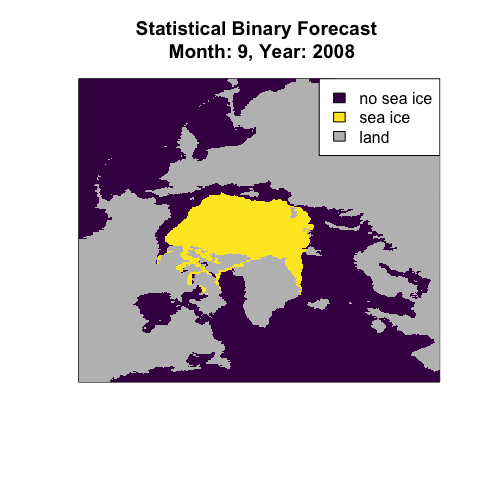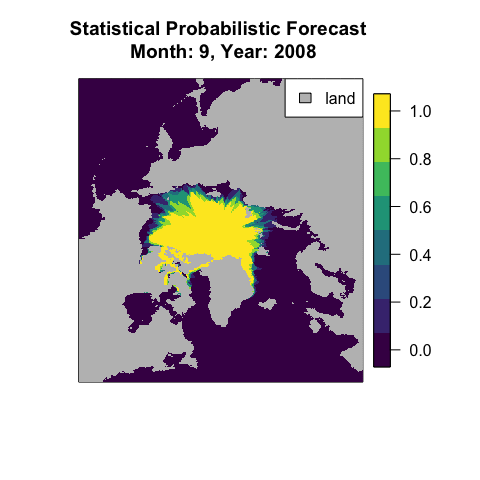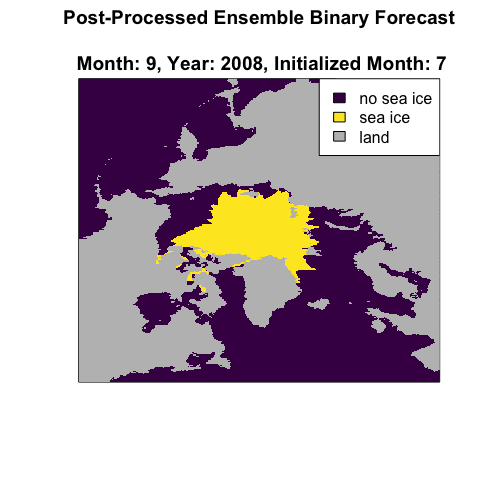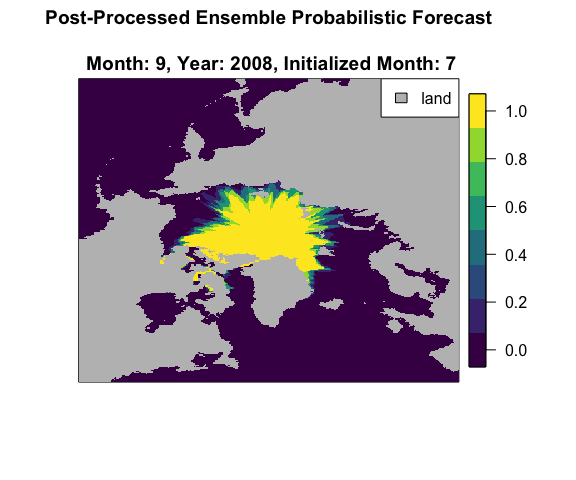Fitting and Generating Contour Forecasts

Introduction

This vignette introduces how to fit and generate sea ice edge contours using the methods proposed in Probabilistic Forecasting of the Arctic Sea Ice Edge with Contour Modeling (Director et al. 2019+). This vignette explains how to fit and generate contours models with the IceCast R package. These forecasts are used as a component in Mixture Contour Forecasts (see the vignette on Mixture Contour Forecasting for more details). To ensure fast loading many values are pre-loaded or only run for a short time. More realistic scripts without these simplifications can be found at https://github.com/hdirector/ProbSeaIce.

In this vignette, we will issue several different forecasts for September 2008. We will use the 25-member ensemble from the European Centre for Medium-Range Weather Forecasts (ECMWF). We have converted the data to a Polar stereographic grid. We will refer to this as the ECMWF forecast. Model output is available from the Copernicus Climate Change Service (2019) and the Sea Ice Prediction Network Predictability Portal. We will also use observations of the monthly sea ice concentration obtained from the National Aeronautics and Space Administration (NASA) satellites Nimbus-7 SMMR and DMSP SSM/I-SSMIS and processed by the bootstrap algorithm. The data are distributed by the National Snow and Ice Data Center (NSIDC) (Comiso 2017).

Setting up

To fit and generate contours, we use the IceCast R package. We’ll also load the viridis and fields packages, since they are useful for visualization.

library("IceCast")
library("viridis")
library("fields")

We also need to specify what month we are interested in forecasting, the start and end years of the training period, the level of minimum sea ice concentration to be counted as containing sea ice, and what year to start fitting the bias correction. This last variable usually corresponds to the earliest year with data available for both observations and predictions.

month <- 9
train_start_year <- 1993
train_end_year <- 2007
level <- 15
train_bc_start_year <- 1993

We also need variables defining how to run the Markov chain Monte Carlo (MCMC) algorithm. This vignette is just for demonstrating how to use the code, so we will choose an extremely low number of iterations. This is NOT enough iterations to use in practice. See the supplemental materials in Director et al. (2019+) for information on determining appropriate chain lengths and burn-in.

n_iter <- 100
burn_in <- 20

Fitting the Contour with MCMC

As a first step for fitting, we load and format the bootstrap sea ice concentration from the binary files. We can load the data as follows where obs_file_path refers to a file path for a folder containing observed bootstrap sea ice concentration data. The observed files must be named using the original file names used by NSIDC (i.e. bt_198301_n07_v02_n.bin). For use with this vignette, the resulting obs object from this set of code is included in the package.

##Not run##
obs_file_path <- 'path_to_observation_data'
bs_version <- 3.1
dat_type_obs <- "bootstrap"
obs <- read_monthly_BS(start_year = train_bc_start_year, end_year = train_end_year,
version = bs_version, file_folder = obs_file_path)

Once we have the observations, we compute their mappings. For more detail on this step, see the section Mapping ice sections in the Contour-Shifting with the IceCast Package vignette. This takes a few minutes, so the resulting obs_maps object has been loaded in the package for use with this vignette.

## Not run ##
obs_maps <- create_mapping(start_year = train_bc_start_year, end_year = train_end_year,
obs_start_year = train_bc_start_year, pred_start_year = NULL,
observed = obs[,month,,], predicted = NULL,
reg_info, month, level, dat_type_obs,
dat_type_pred = NULL, obs_only = TRUE)

We convert the mappings into the line lengths $$y$$ discussed in the paper.

y_obs <- y_obs(maps = obs_maps, reg_info)

We do not fit regions that are completely ice-covered or completely ice-free for all years in the training period. In such cases, our forecast is simply that the region is ice-covered or ice free respectively. Accordingly, we use the y_obs variable to determine which regions need to be fit. We also create a SpatialPolygon object of all fully ice-covered regions that will be included in every generated contour.

temp <- to_fit(y_obs, reg_info)
regs_to_fit <- temp$regs_to_fit full <- temp$full

Next we run the Markov chain Monte Carlo (MCMC) algorithm for all regions. We loop over each region and compute its fit individually.

res <- list()
for (r in regs_to_fit)  {
start_time <- proc.time()
res[[r]] <- fit_cont_pars(r, n_iter, y_obs, reg_info)
end_time <- proc.time()
elapse_time <- end_time - start_time
print(sprintf("MCMC for region %i finished, elapsed time %f", r, elapse_time))
}
##  "MCMC for region 1 finished, elapsed time 9.960000"
##  "MCMC for region 2 finished, elapsed time 0.045000"
##  "MCMC for region 6 finished, elapsed time 1.460000"
##  "MCMC for region 7 finished, elapsed time 0.705000"
##  "MCMC for region 9 finished, elapsed time 0.024000"
##  "MCMC for region 10 finished, elapsed time 0.019000"
##  "MCMC for region 11 finished, elapsed time 0.013000"
##  "MCMC for region 12 finished, elapsed time 0.050000"
##  "MCMC for region 15 finished, elapsed time 0.058000"
##  "MCMC for region 16 finished, elapsed time 0.017000"
##  "MCMC for region 17 finished, elapsed time 0.038000"

Using the results from the MCMC, we can compute the parameters $$\mu$$ and $$\Sigma$$ for each region. All parameters values are stored in a single list called pars. This completes the model fitting.

pars <- list()
for (r in regs_to_fit) {
pars[[r]] <- calc_pars(res[[r]], burn_in, w = res[[r]]$w) } Typically, we store all the information from the model fitting needed to generate a contour in a single list, called gen_info. The information in gen_info is sufficient for generating contours. So, a saved version of this list can can be used to generate additional contours rather than re-estimating the parameters via MCMC. gen_info <- list("regs_to_fit" = regs_to_fit, "full" = full, "pars" = pars, "obs_maps" = obs_maps,"train_start_year" = train_start_year, "train_end_year" = train_end_year) Generating contours Using the gen_info object, we can generate a number of different forecasts of contours. We’ll start with exclusively statistical forecasts to get familiar with the code set up. Then, we’ll consider forecasts that combine the statistical model with dynamic forecasts. These are referred to as post-processed ensemble forecasts in Director et al. (2019+). First, we’ll create a variable specifying the year being forecast. We’ll typically be forecasting the year immediately following the last training year. forecast_year <- gen_info$train_end_year + 1

Statistical Binary Forecast

The first forecast we generate is the statistical binary forecast. This is the contour created by setting each $$y$$ to the $$\mu$$ value estimated from the MCMC. We loop over all regions and generate the contour that fits this description. Note that we have specified stat_only = TRUE to indicate that we are only using statistical information to generate this forecast. Additionally we have specified mean_only = TRUE to indicate that we are only generating a mean contour rather than a distribution of forecasts.

indiv_stat_bin <- list()
for (r in gen_info$regs_to_fit) { indiv_stat_bin[[r]] <- gen_cont(r, pars_r = gen_info$pars[[r]], reg_info,
stat_only = TRUE, mean_only = TRUE)
print(sprintf("stat_bin  region %i contours generated", r))
}
##  "stat_bin  region 1 contours generated"
##  "stat_bin  region 2 contours generated"
##  "stat_bin  region 6 contours generated"
##  "stat_bin  region 7 contours generated"
##  "stat_bin  region 9 contours generated"
##  "stat_bin  region 10 contours generated"
##  "stat_bin  region 11 contours generated"
##  "stat_bin  region 12 contours generated"
##  "stat_bin  region 15 contours generated"
##  "stat_bin  region 16 contours generated"
##  "stat_bin  region 17 contours generated"

We merge the contours generated in each region together into one SpatialPolygon object. We then convert the SpatialPolygon to a binary matrix where each entry is assigned value 1 if its center is ice-covered and 0 otherwise.

stat_bin <- merge_conts(conts = indiv_stat_bin, full = gen_info$full) stat_bin <- conv_to_grid(stat_bin[]) We can then plot the resulting field. Note that for all results in this vignette, the model is only for the Seas of the Arctic. Sea ice forecasts outside this area, categorized as the non-regional ocean by NSIDC, are not forecast. We’ll visualize several forecasts in this vignette, so we first make color variables that can be useed multiple times with the viridis package. n_color <- 8 colors <- viridis(n_color) Finally, we can plot the statistical binary map. stat_bin_vis <- stat_bin #convert na's to a number for visualization (only!) stat_bin_vis[is.na(stat_bin)] <- 1 + 1/n_color image(stat_bin_vis, col = c(colors, "grey"), xaxt = "n", yaxt = "n", main = sprintf("Statistical Binary Forecast \n Month: %i, Year: %i", month, forecast_year)) legend("topright", fill = c(colors, colors[length(colors)], "grey"), legend = c("no sea ice", "sea ice", "land"))Statistical Probabilistic Forecast Using similar code, we can generate a statistical probabilistic forecast. We now set stat_only = TRUE. This is a forecast obtained by generating contours centered at the fitted $$\mu$$ values with the fitted $$\Sigma$$ covariance. Typically, we would generate around 100 contours, but to keep this demo fast, we will just generate 5 contours. We loop over each region and generate 5 contours in each region. n_gen <- 5 indiv_stat_prob <- list() for (r in gen_info$regs_to_fit) {
indiv_stat_prob[[r]] <- gen_cont(r, pars_r = gen_info$pars[[r]], reg_info, n_gen = n_gen, stat_only = TRUE) print(sprintf("stat_prob region %i contours generated", r)) } ##  "stat_prob region 1 contours generated" ##  "stat_prob region 2 contours generated" ##  "stat_prob region 6 contours generated" ##  "stat_prob region 7 contours generated" ##  "stat_prob region 9 contours generated" ##  "stat_prob region 10 contours generated" ##  "stat_prob region 11 contours generated" ##  "stat_prob region 12 contours generated" ##  "stat_prob region 15 contours generated" ##  "stat_prob region 16 contours generated" ##  "stat_prob region 17 contours generated" We then merge the contours generated in each region together to form five SpatialPolygons objects. To obtain a matrix of the estimated probability of each grid box containing sea ice, we apply the prob_map function. The function first uses the conv_to_grid function on each SpatialPolygon to convert it to a binary matrix where each entry is assigned 1 if its center is ice-covered and 0 otherwise. Then, the prob_map function averages the binary fields to estimate the associated probabilities. stat_prob <- merge_conts(conts = indiv_stat_prob, full = gen_info$full)
stat_prob <- prob_map(merged = stat_prob)

We can now plot the statistical probabilistic forecast.

par(oma = c(0, 0, 0, 4))
stat_prob_vis <- stat_prob #convert na's to a number for visualization (only!)
stat_prob_vis[is.na(stat_prob)] <- 1 + 1/n_color
image(stat_prob_vis, col = c(colors, "grey"), xaxt = "n", yaxt = "n",
main = sprintf("Statistical Probabilistic Forecast \n Month: %i, Year: %i",
month, forecast_year))
legend("topright", fill = c("grey"), legend = c("land"))
par(oma = c(0, 0, 0, 1))
image.plot(stat_prob, col = colors, legend.only = TRUE)Post-Processed Ensemble Forecasts

Post-processed ensemble forecasts use information both from the contour model and output from the dynamic ensemble. For demonstration, forecasts of sea ice concentration from the ECMWF dynamic ensemble forecast described in the introduction have been loaded in the package as the object ecmwf_bin.

We’ll focus on the 2.5-month lag (lead time) for this vignette and can compute the resulting initalization month (rounded down for indexing) as follows :

lag <- 2
init_month <- get_init_month(month, lag)

For both post-processed forecasts, we’ll also need to specify to which year the first entry in the ecmwf_bin array corresponds. The data type is set to simple meaning that the entries in ecmwf_bin have value 1 when sea ice is predicted and 0 otherwise.

ecmwf_start_year <- 1993
dat_type_pred <- "simple"

Post-Processed Ensemble Binary Forecast (Contour-Shifting bias correction)

We’ll now consider the binary post-processed ensemble forecast. This is the same as the bias-corrected dynamic model as originally proposed in Director et al. (2017) and modified in Director et al. (2019+). The sea ice edge contour predicted from a dynamic model can be shifted to correct for expected systematic errors through Contour-Shifting. See the Contour-Shifting with the IceCast Package vignette for model details.

We first compute the mappings for the predictions. The pred_maps object is stored in the package for use with this vignette, since this takes a few minutes.

## Not run##
pred_maps <- create_mapping(start_year = train_bc_start_year,
end_year = forecast_year - 1,
obs_start_year = NULL,
pred_start_year = ecmwf_start_year,
observed = NULL, predicted = ecmwf_bin,
reg_info, month, level, dat_type_obs = NULL,
dat_type_pred = "simple", pred_only = TRUE)

We combine mappings for the prediction and for the observations. Note that we have already computed the mapping for the observations when fitting the contour models with MCMC.

maps <- pred_maps
maps$obs_list <- gen_info$obs_maps$obs_list We can apply Contour-Shifting to create a post-processed binary ensemble forecast. As before, we will convert the result to a binary matrix using conv_to_grid. ppe_bin_poly <- contour_shift(maps, predicted = ecmwf_bin[length(ecmwf_start_year:forecast_year),,], bc_year = forecast_year, pred_start_year = ecmwf_start_year, reg_info, level, dat_type_pred) ppe_bin <- conv_to_grid(ppe_bin_poly) Finally, we can plot the resulting forecast ppe_bin_vis <- ppe_bin #convert na's to a number for visualization (only!) ppe_bin_vis[is.na(ppe_bin)] <- 1 + 1/n_color image(ppe_bin_vis, col = c(colors, "grey"), xaxt = "n", yaxt = "n", main = sprintf("Post-Processed Ensemble Binary Forecast \n Month: %i, Year: %i, Initialized Month: %i", month, forecast_year, init_month)) legend("topright", fill = c(colors, colors[length(colors)], "grey"), legend = c("no sea ice", "sea ice", "land"))Post-Processed Ensemble Probabilistic Forecast The last forecast we’ll consider is a post-processed ensemble probabilistic forecast. These forecasts are centered at the bias-corrected dynamic ensemble forecast from the previous section and then contours are generated around this mean contour using the fitted $$\Sigma$$. First, we map the bias-corrected ensemble dynamic forecast for the current forecast year. map_curr <- get_map(ice = ppe_bin_poly, reg_info) Then, we generate contours individually for all regions. The post-processed ensemble erobabilistic forecast is the default model so we do not need to specify any settings. indiv_ppe_prob <- list() for (r in gen_info$regs_to_fit) {
indiv_ppe_prob[[r]] <- gen_cont(r, pars_r = gen_info$pars[[r]], reg_info, n_gen, map_pred_r = map_curr[[r]]) print(sprintf("ppe_prob region %i contours generated", r)) } ##  "ppe_prob region 1 contours generated" ##  "ppe_prob region 2 contours generated" ##  "ppe_prob region 6 contours generated" ##  "ppe_prob region 7 contours generated" ##  "ppe_prob region 9 contours generated" ##  "ppe_prob region 10 contours generated" ##  "ppe_prob region 11 contours generated" ##  "ppe_prob region 12 contours generated" ##  "ppe_prob region 15 contours generated" ##  "ppe_prob region 16 contours generated" ##  "ppe_prob region 17 contours generated" As with the statistical probabilistic forecast, we merge the contours generated for the individual regions together. Then, we use the prob_map function to convert these SpatialPolygon objects to a matrix estimating the probability of sea ice in each grid box. ppe_prob <- merge_conts(conts = indiv_ppe_prob, full = gen_info$full)
ppe_prob <- prob_map(merged = ppe_prob)

Finally, we can plot the resulting contour.

ppe_prob_vis <- ppe_prob #convert na's to a number for visualization (only!)
ppe_prob_vis[is.na(ppe_prob)] <- 1 + 1/n_color
par(oma = c(0, 0, 0, 4))
image(ppe_prob_vis, col = c(colors, "grey"), xaxt = "n", yaxt = "n",
main = sprintf("Post-Processed Ensemble Probabilistic Forecast
\n Month: %i, Year: %i, Initialized Month: %i",
month, forecast_year, init_month))
legend("topright", fill = c("grey"), legend = c("land"))
par(oma = c(0, 0, 0, 1))
image.plot(ppe_prob, col = colors, legend.only = TRUE)